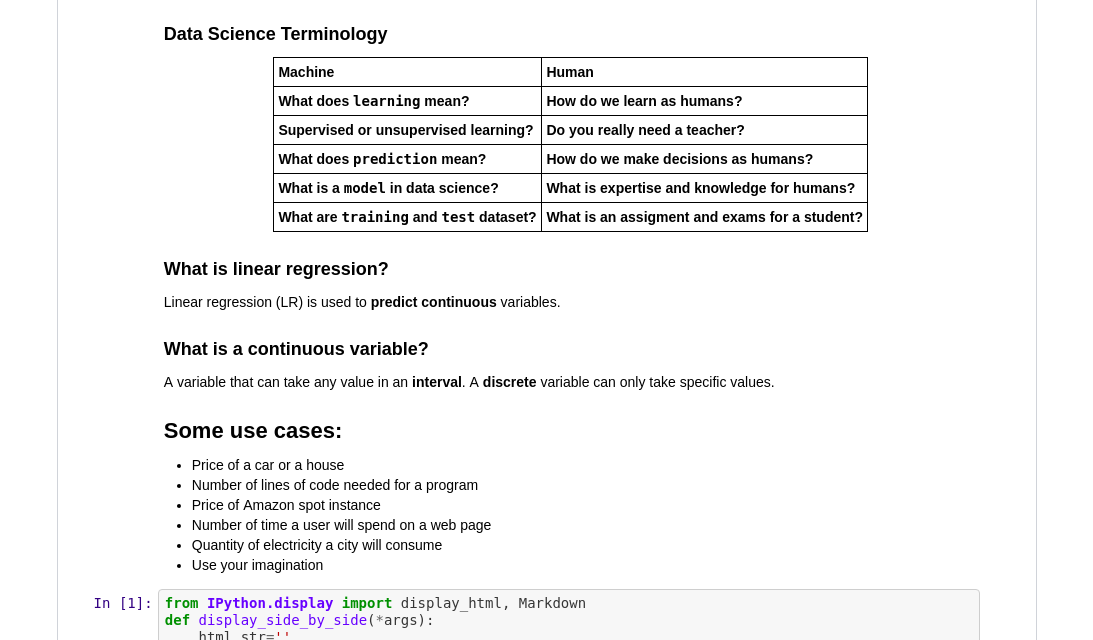25 Jun 2019, 09:04

## Linear Regression - A Tutorial by Thierno Ibrahima

"A learning curve is essential to growth" - Tammy Bjelland

What is linear regression?

"Linear regression is a basic and commonly used type of predictive analysis.

The overall idea of regression is to examine two things:

1. Does a set of predictor variables do a good job in predicting an outcome (dependent) variable?
2. Which variables in particular are significant predictors of the outcome variable, and in what way do they–indicated by the magnitude and sign of the beta estimates–impact the outcome variable?

These regression estimates are used to explain the relationship between one dependent variable and one or more independent variables. The simplest form of the regression equation with one dependent and one independent variable is defined by the formula y = c + b*x, where y = estimated dependent variable score, c = constant, b = regression coefficient, and x = score on the independent variable. " - Statistics Solutions. (2013). What is Linear Regression

Thierno Ibrahima looks at linear regression and how to implement it from scratch using python.

See his tutorial on Linear Regression on his Github!Thierno IbrahimaThierno is a data engineer from Dakar, Senegal. He is a co founder of galsenAI a senegalease community in artificial intelligence with more than 700 members on meetups.

Learn how to use open data from africa at their next meetup in July 2019.